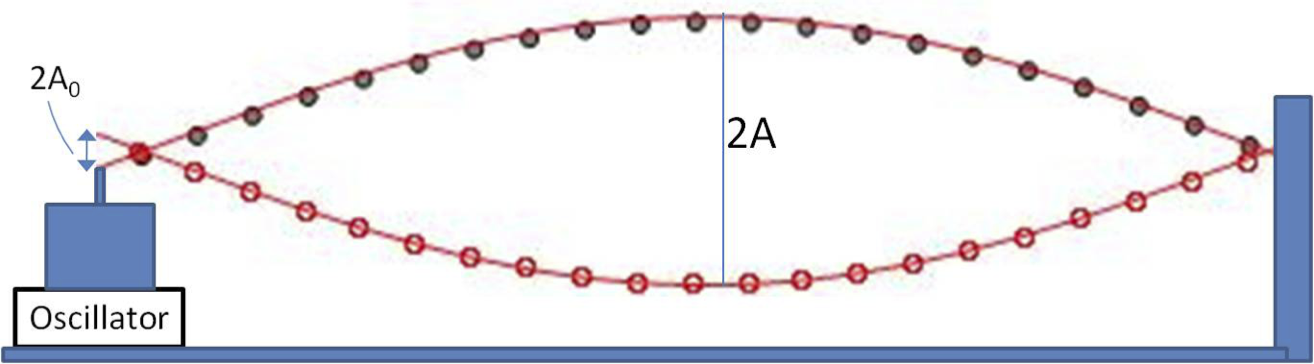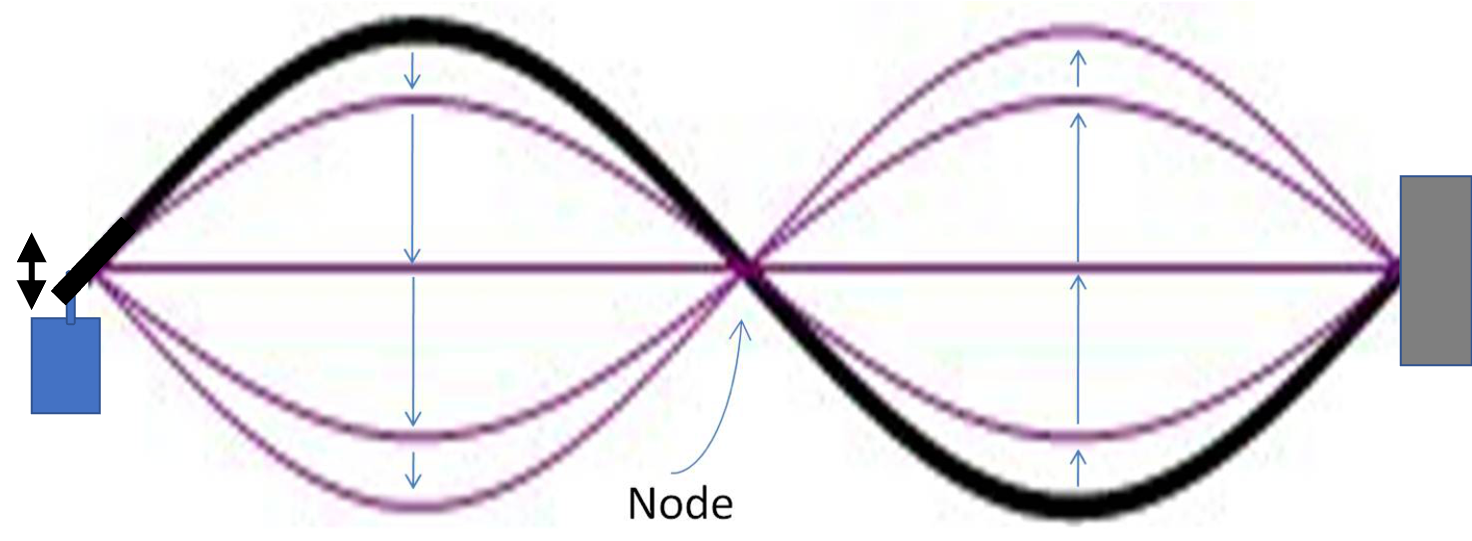## Section14.4Resonance of a String

One way of vibrating a stretched string in one of the normal modes is to pull the string to match the shape of the normal mode profile, and then release the deformed string from rest. The subsequent motion of the string will be the oscillatory motion of that mode. Although this method will work, but it is very difficult to achieve.

A better way to excite a string in a normal mode oscillation is to use resonance and drive the string into the normal mode. In the case of a simple harmonic oscillator, we had only one frequency to excite. Here, we have a large number of normal frequencies, and we can excite any one of them by driving at the frequency of that normal mode. To do that we attach the string to an oscillator near a node of the corresponding normal mode as shown in Figure 14.4.1 and then let vibrate the oscillator at the frequency of he mode.Figure 14.4.1. Generation of standing wave by driving vibrations by an oscillator near the node of the fundamental normal mode.

If we vibrate the string at the second normal mode of the string, we will excite the string to vibrate in this mode as illustrated in Figure 14.4.2.Figure 14.4.2. Driving resonance of the second normal mode of a string.

This experimental situation presents the following conditions on the ends of the string, called boundary conditions. With $y$ for the displacement, $x$ axis horizontal with origin at the node, the condition on the vibration function will be

\begin{align} \amp y(x,t)|_{x=-\epsilon} = A_0\ cos(\omega t),\label{eq-boundary-cond-1}\tag{14.4.1}\\ \amp y(x,t)|_{x=L} = 0.\label{eq-boundary-cond-2}\tag{14.4.2} \end{align}

Here $\epsilon \lt\lt \lambda$ and you can think of vibrator being “at the node”. When frequency $\omega$ of the driver is near the frequency of a normal mode, the amplitude $A$ is much larger than $A_0\text{,}$ which is the amplitude of the tip of the vibrator, due to resonance.

The function that describes the the wave on the string in a normal mode

\begin{equation} y(x,t) = A\sin(k_n x - \omega_n t),\label{eq-driving-fundamental-mode-string-1}\tag{14.4.3} \end{equation}

where $\omega_n$ is the angular frequency and $k_n$ the wavenumber of the mode. In Figure 14.4.1>, $n=1$ with

\begin{equation*} k_1 \approx 2L,\ \ \omega_1 = v\,k_1,\ \ v=\sqrt{T/\mu}, \end{equation*}

where $T$ is the tension and $\mu$ is mass per unit length in the string. Other modes are related to this fundamental by $\omega_n = n \omega_1$ and $k_n = n k_1\text{.}$

In Figure 14.4.1, Eq. (14.4.1) tells us that amplitude of the tip of oscillator will be related to the amplitude of the wave generated and the location of the node. Suppose, the mode is at its highest at $t=0\text{,}$ then the tip will fall on the mode function given in Eq. (14.4.3) at $x=-\epsilon$ and $t=0\text{.}$

\begin{equation} A\sin(k_n \epsilon) = A_0.\tag{14.4.4} \end{equation}

In Figure 14.4.1, we have mode $n=1\text{.}$ This gives

\begin{equation} A = \dfrac{A_0}{\sin(k_n\epsilon)} = \dfrac{A_0}{ \sin( \pi\epsilon/L)}.\tag{14.4.5} \end{equation}

As an example from lab, let's say $\epsilon=2.0\text{ cm}\text{,}$ $A_0=1.0\text{ cm}\text{,}$ $L=100.0\text{ cm}\text{.}$ Then, the amplitue of the standing wave generated will be $A = 16\text{ cm}\text{.}$

A Nylon guitar string has diameter $1.33\text{ mm}$ and length $65\text{ cm}\text{.}$ The density of Nylon is $1.14\text{ g/cm}^3\text{.}$ The string is tightened to a tension of $100\text{ N}\text{.}$ What are the frequencies of three lowest frequency modes that you can excite the string into?

Hint

Use $f\lambda = v =\sqrt{T/\mu}\text{.}$ Find $\mu$ from regular density $\rho$ by using total mass of the string to find the relation $\mu = \rho A\text{,}$ where $A$ is the area of cross section. Also make sure you use a consistent set of units.

$194\text{ Hz}\text{,}$ $387\text{ Hz}\text{,}$ $581\text{ Hz}\text{.}$

Solution

The wavelengths of the lowest three modes will be $\lambda_1=2L\text{,}$ $\lambda_2=L\text{,}$ and $\lambda_3=2L/3\text{.}$ Lets write these as $\lambda_n = 2L/n\text{.}$ Then, the frequency of the three lowest modes will be

\begin{equation*} f_n = \frac{v}{\lambda_n} = \frac{nv}{2L} = n f_1, \text{ with } f_1 = \frac{v}{2L}, \end{equation*}

where $v$ is the wave speed, which is related to the tension $T$ and linear density $\mu\text{.}$

\begin{equation*} v = \sqrt{\frac{T}{\mu}}. \end{equation*}

Let $\rho$ denote the density, i.e., mass per unit volume, and $A$ the area of cross-section of the string. Equating the total mass of string using linear density and regular density will give us the relation from which we can compute the linear density. Let $m$ denote the total mass and $V=AL$ the total volume.

\begin{equation*} m = \mu L,\ \ \ m = \rho V = \rho A L. \end{equation*}

Therefore,

\begin{equation*} \mu = \rho A. \end{equation*}

Here $A=\pi R^2 = \pi D^2/4\text{,}$ where $D$ is the diameter. Numerically

\begin{equation*} A = \frac{\pi}{4} (1.33\times10^{-3})^2 = 1.389\times 10^{-6}\text{ m}^2. \end{equation*}

Therefore,

\begin{equation*} \mu = 1140 \text{ kg/m}^3 \times 1.389\times 10^{-6}\text{ m}^2 = 1.58\times 10^{-3}\text{ kg/m}. \end{equation*}

We use this to get $v\text{.}$

\begin{equation*} v = \sqrt{\frac{100\text{ N}}{1.58\times 10^{-3}\text{ kg/m}}} = 251.6\text{ m/s}. \end{equation*}

Therefore, the three lowest frequencies are

\begin{align*} \amp f_1 = \frac{v}{2L} = \frac{251.6}{2\times 0.65} = 194\text{ Hz}. \\ \amp f_2 = 2 \times f_1 = 387\text{ Hz}. \\ \amp f_3 = 3 \times f_1 = 581\text{ Hz}. \end{align*}